Minima/ maxima (statement questions) - Geometry questions

Chapter 6 Class 12 Application of Derivatives
Concept wise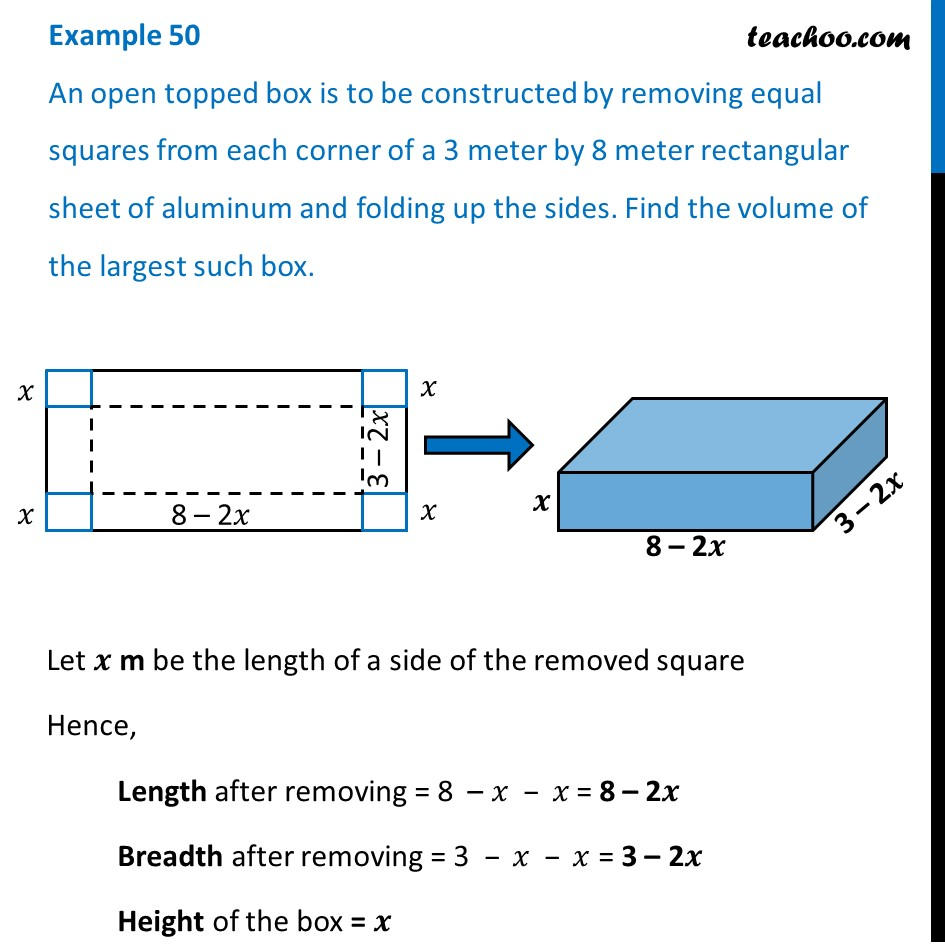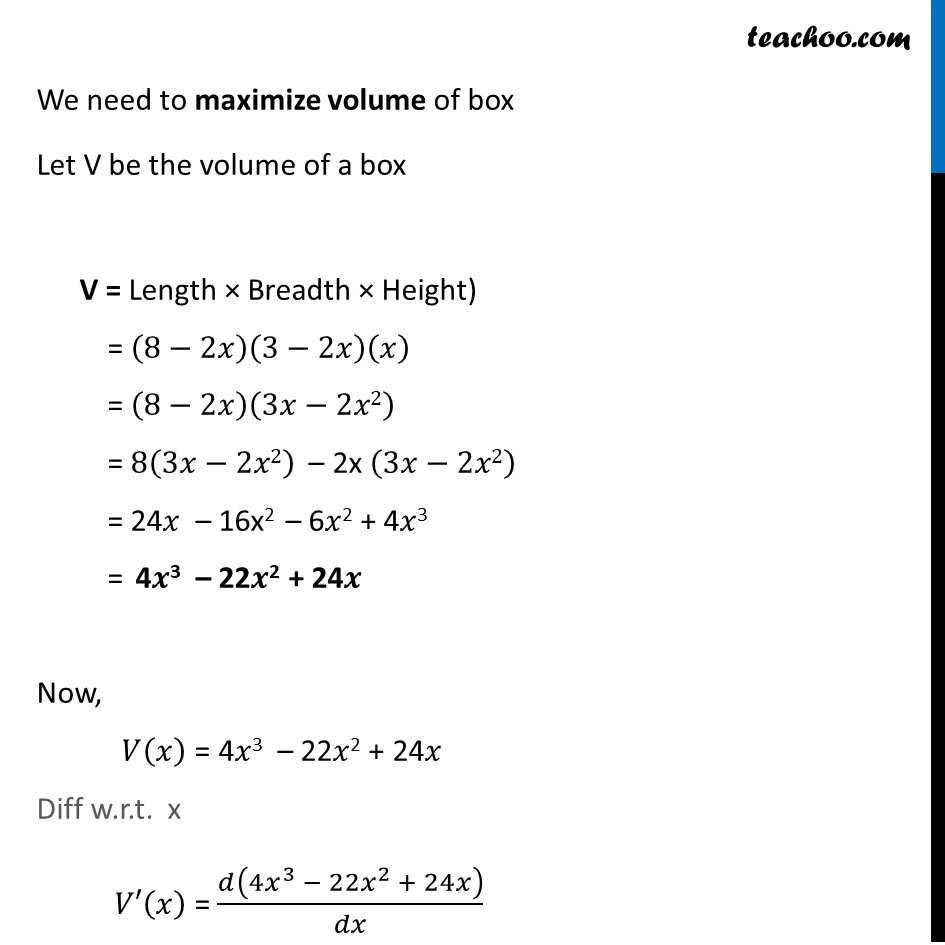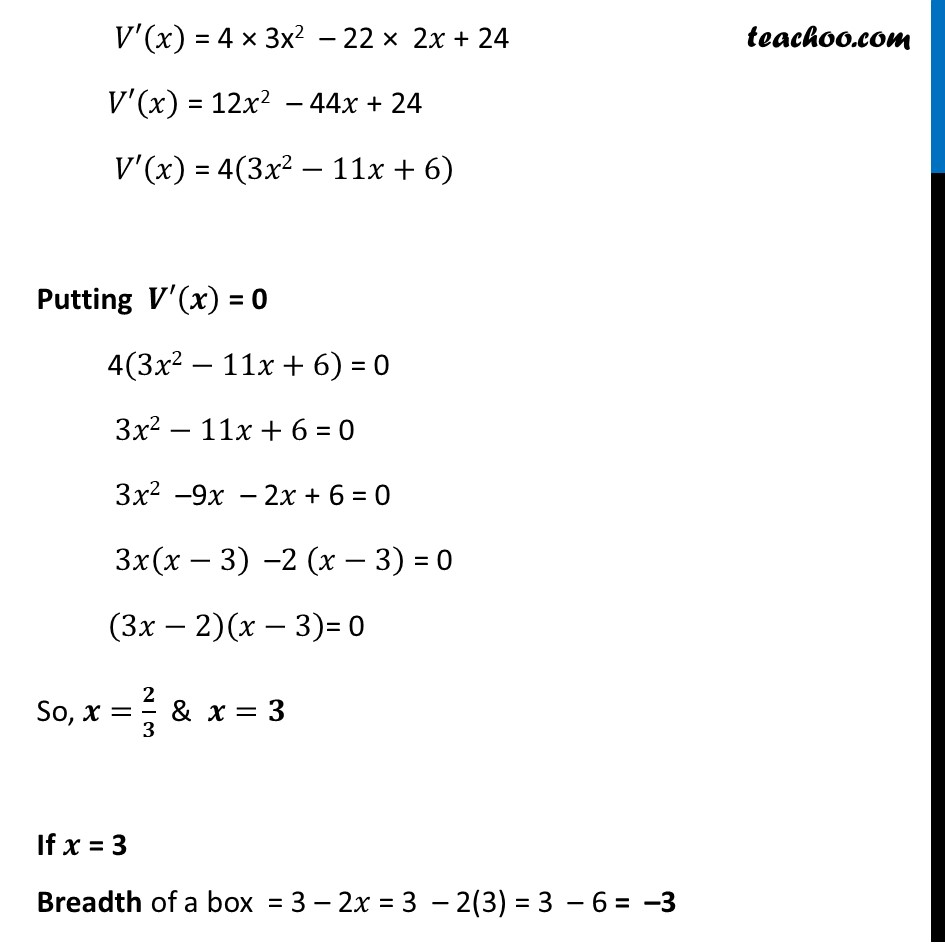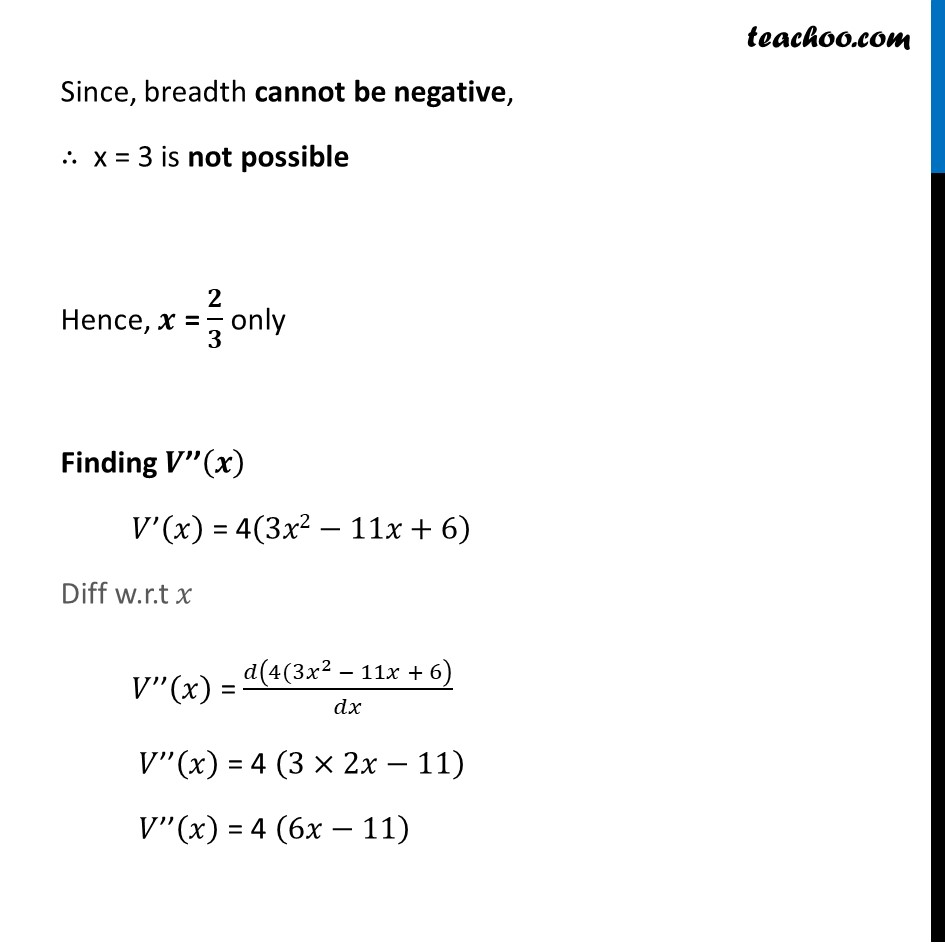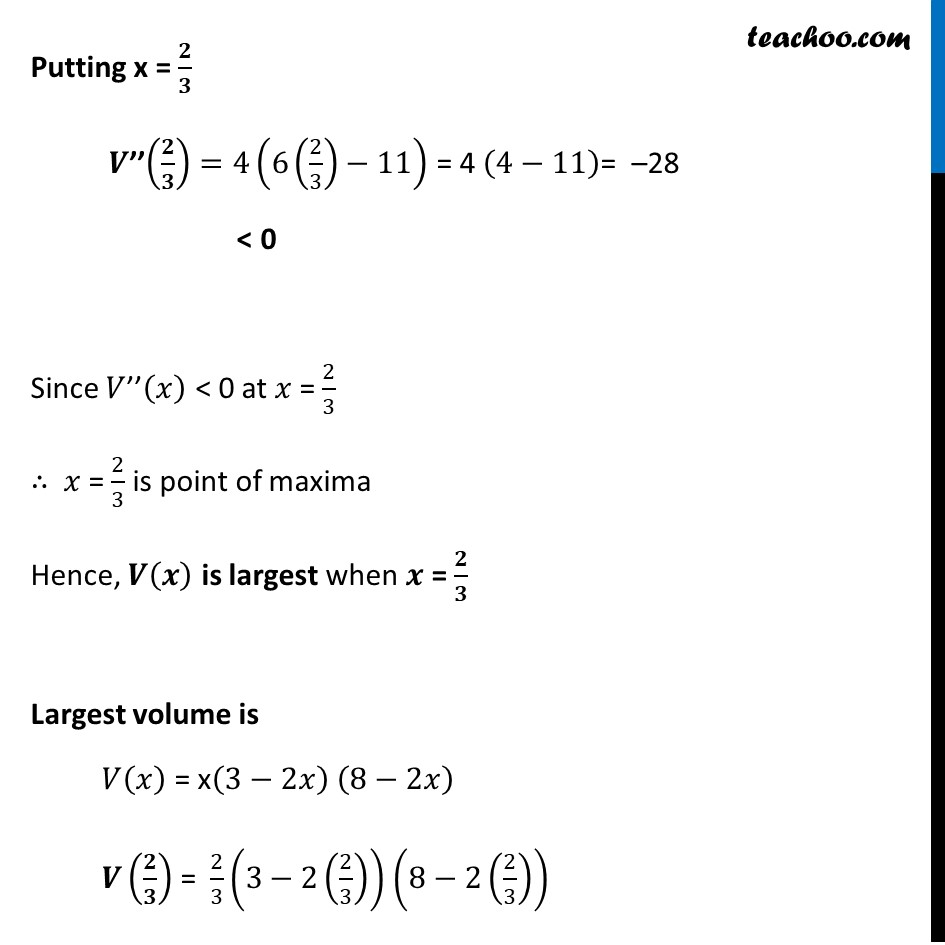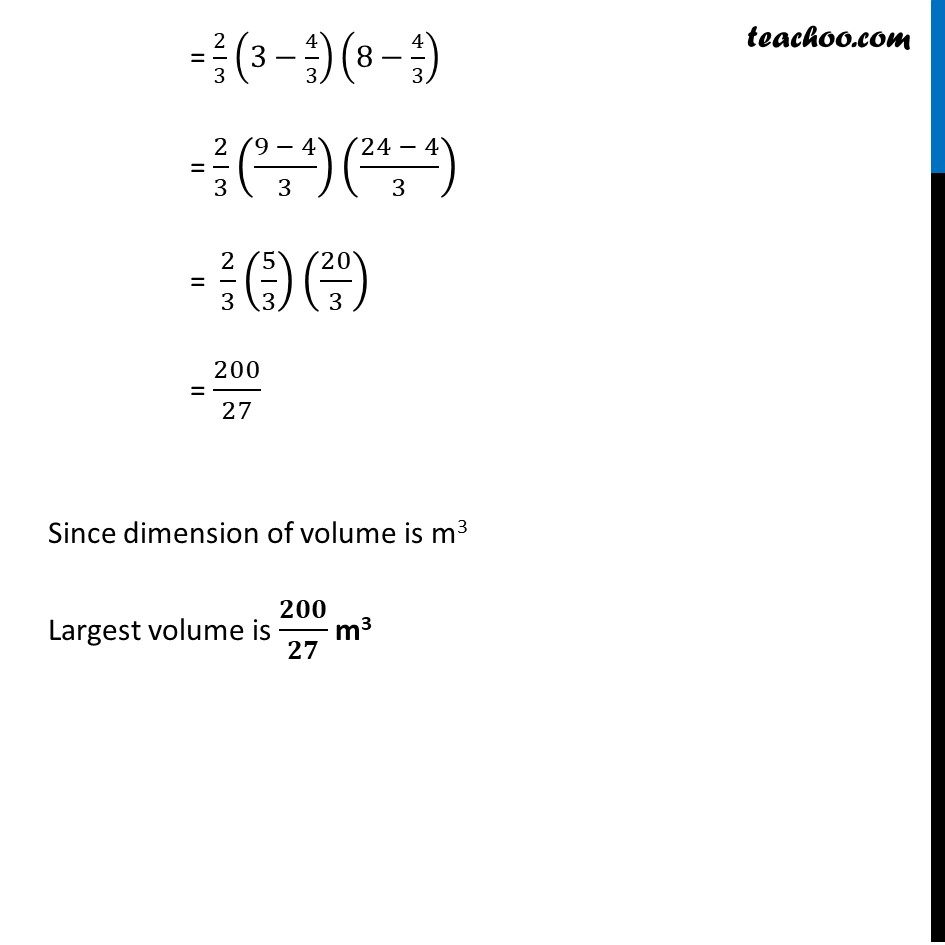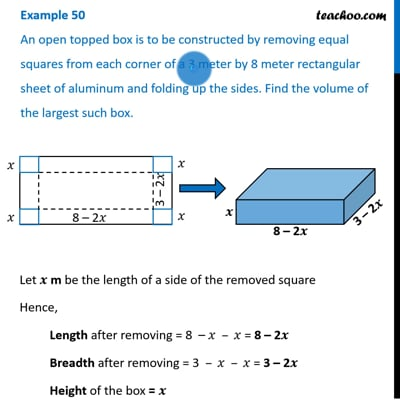This video is only available for Teachoo black users

Introducing your new favourite teacher - Teachoo Black, at only ₹83 per month

### Transcript

Example 50 An open topped box is to be constructed by removing equal squares from each corner of a 3 meter by 8 meter rectangular sheet of aluminum and folding up the sides. Find the volume of the largest such box.Let 𝒙 m be the length of a side of the removed square Hence, Length after removing = 8 – 𝑥 – 𝑥 = 8 – 2𝒙 Breadth after removing = 3 – 𝑥 – 𝑥 = 3 – 2𝒙 Height of the box = 𝒙 We need to maximize volume of box Let V be the volume of a box V = Length × Breadth × Height) = (8−2𝑥)(3−2𝑥)(𝑥) = (8−2𝑥)(3𝑥−2𝑥2) = 8(3𝑥−2𝑥2) – 2x (3𝑥−2𝑥2) = 24𝑥 – 16x2 – 6𝑥2 + 4𝑥3 = 4𝒙3 – 22𝒙2 + 24𝒙 Now, 𝑉(𝑥) = 4𝑥3 – 22𝑥2 + 24𝑥 Diff w.r.t. x 𝑉′(𝑥) = 𝑑(4𝑥^3 − 22𝑥^2 + 24𝑥)/𝑑𝑥 𝑉′(𝑥) = 4 × 3x2 – 22 × 2𝑥 + 24 𝑉′(𝑥) = 12𝑥2 – 44𝑥 + 24 𝑉′(𝑥) = 4(3𝑥2−11𝑥+6) Putting 𝑽′(𝒙) = 0 4(3𝑥2−11𝑥+6) = 0 3𝑥2−11𝑥+6 = 0 3𝑥2 –9𝑥 – 2𝑥 + 6 = 0 3𝑥(𝑥−3) –2 (𝑥−3) = 0 (3𝑥−2)(𝑥−3)= 0 So, 𝒙=𝟐/𝟑 & 𝒙=𝟑 If 𝒙 = 3 Breadth of a box = 3 – 2𝑥 = 3 – 2(3) = 3 – 6 = –3 Since, breadth cannot be negative, ∴ x = 3 is not possible Hence, 𝒙 = 𝟐/𝟑 only Finding 𝑽’’(𝒙) 𝑉’(𝑥) = 4(3𝑥2−11𝑥+6) Diff w.r.t 𝑥 𝑉’’(𝑥) = 𝑑(4(3𝑥^2 − 11𝑥 + 6)/𝑑𝑥 𝑉’’(𝑥) = 4 (3×2𝑥−11) 𝑉’’(𝑥) = 4 (6𝑥−11) Putting x = 𝟐/𝟑 𝑽’’(𝟐/𝟑)=4(6(2/3)−11) = 4 (4−11)= –28 < 0 Since 𝑉’’(𝑥) < 0 at 𝑥 = 2/3 ∴ 𝑥 = 2/3 is point of maxima Hence, 𝑽(𝒙) is largest when 𝒙 = 𝟐/𝟑 Largest volume is 𝑉(𝑥) = x(3−2𝑥) (8−2𝑥) 𝑽(𝟐/𝟑) = 2/3 (3−2(2/3)) (8−2(2/3)) = 2/3 (3−4/3)(8−4/3) = 2/3 ((9 − 4)/3)((24 − 4)/3) = 2/3 (5/3)(20/3) = 200/27 Since dimension of volume is m3 Largest volume is 𝟐𝟎𝟎/𝟐𝟕 m3